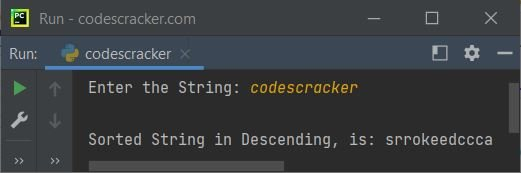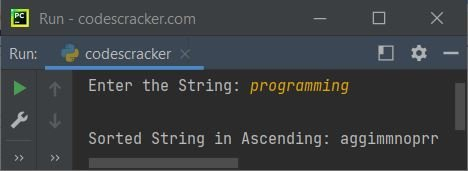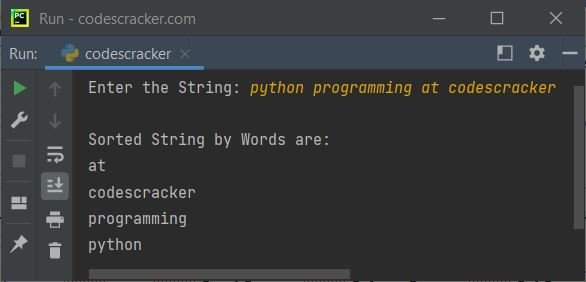# Python Program to Sort String in Alphabetical Order

This article contains multiple programs in Python, that sorts string, entered by user at run-time of the program. List of programs covered in this article:

• Sort string in ascending order using sorted() method
• Sort string in descending order using sorted() method
• Sort string without using sorted() method
• Sort string by words in alphabetical order

Either ascending or descending, the string gets sorted alphabetically.

## Python Sort String in Ascending Order

The question is, write a Python program to sort a string in ascending order. The string must be received by user at run-time. The program given below is its answer:

```print("Enter the String: ", end="")
str = input()

str = sorted(str)
str = ''.join(str)

print("\nSorted String is:", str)```

The snapshot given below shows the sample run of above Python program, with user input codescracker as string to sort and print the sorted string in ascending order, by alphabetical wise:In above program, the sorted() method sorts the string alphabetically. But the string gets sorted and converted in the form of list. That is, if you remove the following statement from the above program:

`str = ''.join(str)`

Then the output would be:

`Sorted String is: ['a', 'c', 'c', 'c', 'd', 'e', 'e', 'k', 'o', 'r', 'r', 's']`

So the join() method used in above program, to take all the items of an iterable and join them into one string.

## Python Sort String in Descending Order

This program sorts a given string in descending order. The only change I've done in this program, in compare to previous, is that the addition of second argument to sorted() method. That argument is `reverse=True`. Rest of all the codes remains same.

```print("Enter the String: ", end="")
str = input()

str = sorted(str, reverse=True)
str = ''.join(str)

print("\nSorted String in Descending, is:", str)```

Here is its sample run, with same user input as of previous program's sample run:## Python Sort a String without using sorted() Function

Here is another program in Python, used to sort a given string in ascending order, but without using sorted() method:

```print("Enter the String: ", end="")
str = input()

strLen = len(str)
for i in range(strLen):
for j in range(strLen-1):
if str[j] > str[j+1]:
str = str[:j] + str[j+1] + str[j] + str[j+2:]

print("\nSorted String in Ascending:", str)```

Sample run of above program, with user input programming is shown in the snapshot given below:Since the string objects are immutable in Python, therefore we can not perform initialization by index wise, to a string. Therefore I've done the slicing to do the job. In above program, the following statement:

`str = str[:j] + str[j+1] + str[j] + str[j+2:]`

basically tells that the character at index j gets interchanged with the character at index j+1.

## Python Sort Words in Alphabetical Order

This is the last program of this article, created to sort string by words, in of course, alphabetical order:

```print("Enter the String: ", end="")
str = input()

words = []
for word in str.split():
words.append(word.lower())

words.sort()
print("\nSorted String by Words are:")
for word in words:
print(word)```

Here is its sample run with user input python programming at codescracker as string to sort word-wise:#### Same Program in Other Languages

Python Online Test

« Previous Program Next Program »